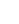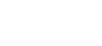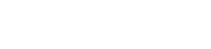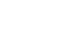# Calculate Capacity of Pile Group and EfficiencyReading time: 1 minute

Capacity of pile group is the sum of the individual capacities of piles, but it is influenced by the spacing between the piles. Piles are driven generally in groups in regular pattern to support the structural loads. The structural load is applied to the pile cap that distributes the load to individual piles. If piles are spaced sufficient distance apart, then the capacity of pile group is the sum of the individual capacities of piles. However, if the spacing between piles is too close, the zones of stress around the pile will overlap and the ultimate load of the group is less than the sum of the individual pile capacities especially in the case of friction piles, where the efficiency of pile group is much less.

## Capacity of Pile Group

Group action of piles is evaluated by considering the piles to fail as a unit around the perimeter of the group. Both end bearing and friction piles are considered in evaluating the group capacity. End bearing pile is evaluated by considering the area enclosed by the perimeter of piles as the area of footing located at a depth corresponding to the elevation of pile tips. The friction component of pile support is evaluated by considering the friction that can be mobilized around the perimeter of the pile group over the length of the piles as shown in figure below:Pile group capacity, Q = q0 X B2 + 4 x B x L x f (Square) Where, Q = ultimate capacity of pile group q0 = ultimate bearing pressure of footing of area B2 (B = size of pile group) L = Length of pile f = shear resistance

## Efficiency of Pile Group

The efficiency of pile group depends on the following factors:
1. Spacing of piles
2. Total number of piles in a row and number of rows in a group, and
3. Characteristics of pile (material, diameter and length)
The reduction in total bearing value of group of piles is more in case of friction piles, particularly in clayey soils. No reduction in grouping occurs in end bearing piles. The pile groups which are resisting the load by combined action of friction and end bearing, only the load carrying capacity of friction is reduced. The efficiencyof the pile group can be calculated by using the following formula:Thus, the pile group efficiency is equal to the ratio of the average load per pile in the group at which the failure occurs to the ultimate load of a comparable single pile. Efficiency of a pile group can also be obtained by using Converse - Labarre formula:Where m = number of rows n = number of piles in a rowin degrees d = diameter of pile end s = spacing of piles. Generally center to center spacing between piles in a group is kept between 2.5 d and 3.5d where d is the diameter of the pile. Read More on Pile Foundations